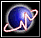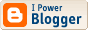Yet another weird SF fan
 I'm a mathematician, a libertarian, and a science-fiction fan. Common sense? What's that?Go to first entry

## Archives

<< current

 Yet another weird SF fan

### Another Way to Look at the Collatz Problem

The Collatz problem can be summarized as follows.

First, start with a number n and carry out the following transformation over and over:

• If the number is odd. multiply it by 3 and add 1.
• If the number is even, divide it by 2.
This will produce a sequence. For example, if n = 7, the sequence will become:
7 22 11 34 17 52 26 13 40 20 10 5 16 8 4 2 1 4 2 1 4 2 1 …

In the case where n = 7, the sequence eventually arrives at a 4 2 1 … cycle. In all known cases, starting from a positive whole number, the sequence will arrive at the same cycle. The Collatz problem is: Are there any exceptions?

#### My idea

It might be possible to assign an ordinal number to each integer so that dividing an even number by two or applying 3n + 1 to an odd number greater than 1 will decrease the corresponding ordinal. Since any decreasing sequence of ordinals must be finite, the sequence must reach 1 eventually.

I have not been able to make this work.

 Profiles My Blogger ProfileX-treme Tracker The Atom Feed